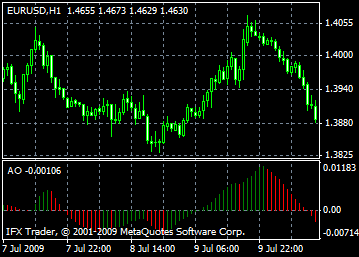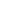# Awesome Oscillator - AO

Bill Williams’ Awesome Oscillator (AO) is a 34-period moving average built on midpoints of bars (H+L)/2. All this is subtracted from the 5-period simple moving average created on midpoints of bars (H+L)/2. It reflects accurately what happens currently, i.e. shows what happens with the market’s driving force.

Saucer

The Saucer is considered to be the only buy signal that arises when the histogram is above zero line. You should remember that the Saucer is formed when the histogram is rebuilt, thus a downtrend changes to an uptrend. The second column is colored red and is below the first one, while the third column is higher than the second one and is colored green.

You should always keep in mind when using the Saucer buy signal that all the AO columns should be higher than the zero line.

Zero Line Cross

Buy signal is created when the histogram leaves negative zone and approaches the positive one. However, to create this signal, only two columns are needed.

The first column should be lower than the zero level and the second one should cross it (transfer from negative to positive reading). Simultaneous existence of buy and sell signals is impossible.

Twin Peaks

It is the only buy signal that may appear when the histogram figures are lower than the zero mark.

You should understand that the signal will occur if you have a downward peak (the lowest mark) below the zero line, and then another downward peak (the smallest by absolute value negative reading near the zero level) that is higher than the first one.

What is more, the histogram should be below the zero line. If it breaks the zero mark between two peaks, the buy signal is useless: the Zero Line Cross buy signal is forming.

Another important moment is that every next histogram’s peak should be higher (the smallest by absolute value negative number closest to the zero line) than the previous one.

In case when an extra peak is developing, which is even higher (closer to the zero line), and the histogram still does not cross the zero line, an additional buy signal will be formed.

Sell signals

The AO’s sell signals and buy signals are alike. For example, the Saucer signal is reversed and below zero. The Zero Line Cross is formed by descending, i.e. the first column is above zero; and the second one, below it. The Twin Peaks signal is above the zero level and also reversed.## Calculation

AO is a 34-period simple moving average, plotted through the central points of the bars (H+L)/2, and subtracted from the 5-period simple moving average, graphed across the central points of the bars (H+L)/2.

MEDIAN PRICE = (HIGH+LOW)/2

AO = SMA(MEDIAN PRICE, 5)-SMA(MEDIAN PRICE, 34)

where

SMA - Simple Moving Average.

All MT5 content